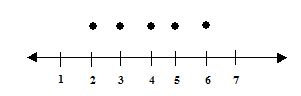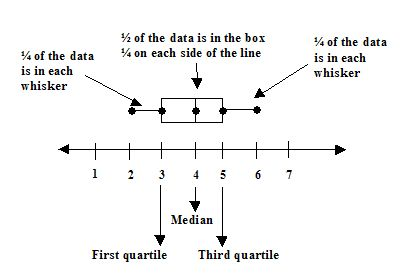Email us to get an instant 20% discount on highly effective K-12 Math & English kwizNET Programs!

#### Online Quiz (WorksheetABCD)

Questions Per Quiz = 2 4 6 8 10

### Grade 7 - Mathematics10.2 Box-and-Whisker Plot

• Central tendency describes the middle or a typical value of the data set and is measured using the mean, median, and mode.
• Variability describes how spread out the data is.
• Quartiles divide a data set into four equal parts. The third quartile minus the first quartile is the range for the middle half of the data.
• A box-and-whisker plot shows the distribution of data. The middle half of the data is represented by a box with a vertical line at the median. the lower first and upper fourth are represented by whiskers that extend to the smallest and largest values.
Example:
Draw a box-and-whisker plot for the table below, that summarizes a veterinaian's record for kitten litters born in a given year.

 Litter Size 2 3 4 5 6 Number of Litters 1 6 8 11 1

1. Order the data and find the smallest value, first quartile, median, third quartile and largest value.
 2 3 3 3 3 3 3 4 4 4 4 4 4 4 4 5 5 5 5 5 5 5 5 5 5 5 6

 Lower half Upper half 2 3 3 3 3 3 3 4 4 4 4 4 4 4 4 5 5 5 5 5 5 5 5 5 5 5 6 First quartile Second quartile Third quartile median of lower half:3 Median:4 median of upper half:5

smallest value: 2
first quartile: 3
median: 4
third quartile: 5
largest value: 6

2. Draw a number line and plot above each value from step 13. Draw the box and whiskers.Directions: Answer the following questions. Also write at least 5 examples of your own.
 Q 1: The _____ quartile is the middle (the median) of the lower half of the data. secondfirstthird Q 2: _________ of the data lies below the first quartile and three-fourths lies above. (the 25th percentile)One-fourthTwo-thirdsOne-half Q 3: Find the third quartile of the following data: 85,92,78,88,90,88,89Answer: Q 4: Find the first quartile of the following data: 85,92,78,88,90,88,89Answer: Q 5: Find the first quartile of the following data: 13,14,16,18,18,21,12,21,11,19,15,13Answer: Q 6: _______ of data set is the second quartile of data set.MedianModeMean Question 7: This question is available to subscribers only! Question 8: This question is available to subscribers only!

#### Subscription to kwizNET Learning System offers the following benefits:

• Unrestricted access to grade appropriate lessons, quizzes, & printable worksheets
• Instant scoring of online quizzes
• Progress tracking and award certificates to keep your student motivated
• Unlimited practice with auto-generated 'WIZ MATH' quizzes